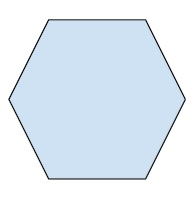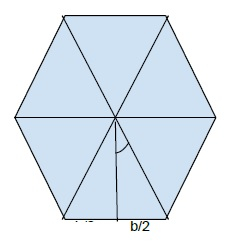# Apothem of a n-sided regular polygon in C++

n-sided regular polygon is a closed figure of n sides that has all sides and angles of equal length. The below figure shows a 6 sided regular polygon commonly known as hexagon.Apothem is a line in the polygon that connects the center of the figure to the side. And it is perpendicular to one of its sides that makes it smallest in length.

Now, let drive the formula for its length.

The angle made by the side of an n sided polygon is 360/n.Now, as in the figure, the angle is equal to (360 / n )/2 = 180 /n

Now taking the triangle, we can conclude that

tan ø = b/2 / h = b/2h
2h * tan ø = b
h = b/2*tanø , ø = 180 /n
h = b/2*tan(180/n)

Let's implement this to a program to find the length of apothem when the number of sides and length of each side of the polygon are given.

## Formula

Given n = number of side , b = length of each side.
h = length of apothem,
h = b/2 * tan(180/n)

## Example

Live Demo

#include<iostream>
#include <math.h>
using namespace std;
void apothemLength(int n, float a){
if (a < 0 && n < 0)
cout<<"invalid values";
else
cout<<"the length of apothem = "<< (a/(2*tan((180/n)*3.14159/180)));
}
int main(){
float a = 12;
int n = 9;
apothemLength(n, a);
return 0;
}

## Output

the length of apothem = 16.4849